JEE  >  Test: Vector Algebra- 2

# Test: Vector Algebra- 2 - JEE

Test Description

## 25 Questions MCQ Test Mathematics (Maths) Class 12 - Test: Vector Algebra- 2

Test: Vector Algebra- 2 for JEE 2023 is part of Mathematics (Maths) Class 12 preparation. The Test: Vector Algebra- 2 questions and answers have been prepared according to the JEE exam syllabus.The Test: Vector Algebra- 2 MCQs are made for JEE 2023 Exam. Find important definitions, questions, notes, meanings, examples, exercises, MCQs and online tests for Test: Vector Algebra- 2 below.
Solutions of Test: Vector Algebra- 2 questions in English are available as part of our Mathematics (Maths) Class 12 for JEE & Test: Vector Algebra- 2 solutions in Hindi for Mathematics (Maths) Class 12 course. Download more important topics, notes, lectures and mock test series for JEE Exam by signing up for free. Attempt Test: Vector Algebra- 2 | 25 questions in 25 minutes | Mock test for JEE preparation | Free important questions MCQ to study Mathematics (Maths) Class 12 for JEE Exam | Download free PDF with solutions
 1 Crore+ students have signed up on EduRev. Have you?
Test: Vector Algebra- 2 - Question 1

### Zero Vector

Detailed Solution for Test: Vector Algebra- 2 - Question 1

A vector whose initial and terminal points coincides is called Zero Vector. The magnitude of Zero Vector is also Zero.

Test: Vector Algebra- 2 - Question 2

### If a vector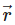is expressed in component form as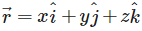then the magnitude of the vectoris

Detailed Solution for Test: Vector Algebra- 2 - Question 2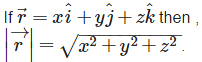Test: Vector Algebra- 2 - Question 3

### Find the value of x for which x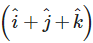is a unit vector

Detailed Solution for Test: Vector Algebra- 2 - Question 3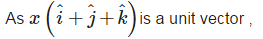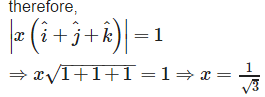Test: Vector Algebra- 2 - Question 4

Find the angle between two vectors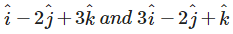Detailed Solution for Test: Vector Algebra- 2 - Question 4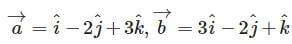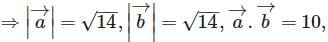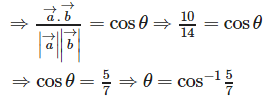Test: Vector Algebra- 2 - Question 5

Find λ and μ if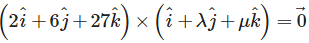Detailed Solution for Test: Vector Algebra- 2 - Question 5

It is given that: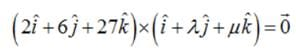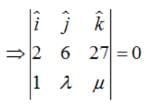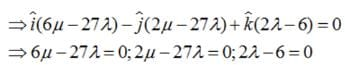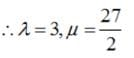Test: Vector Algebra- 2 - Question 6

Unit Vector is

Detailed Solution for Test: Vector Algebra- 2 - Question 6

The vector whose magnitude is always 1 or unity is called a Unit Vector.

Test: Vector Algebra- 2 - Question 7

If a vector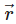is expressed in component form as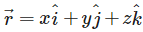then x, y and z are referred to as

Detailed Solution for Test: Vector Algebra- 2 - Question 7

Inx , y and z represents the components ofalong x-axis ,y-axis and z-axis respectively.

Test: Vector Algebra- 2 - Question 8

Show that the points A(1, – 2, – 8), B (5, 0, – 2) and C (11, 3, 7) are collinear, and find the ratio in which B divides AC.

Detailed Solution for Test: Vector Algebra- 2 - Question 8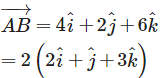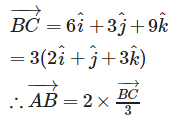Therefore , AB and BC are parallel, but point B is common , so points , A,B,C are collinear. As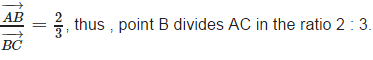Test: Vector Algebra- 2 - Question 9

Find the projection of the vector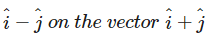Detailed Solution for Test: Vector Algebra- 2 - Question 9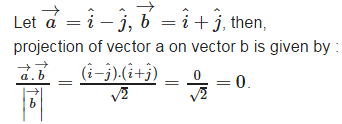Test: Vector Algebra- 2 - Question 10

Let the vectors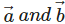be such that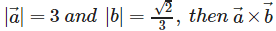is a unit vector if the angle between vectorsis

Detailed Solution for Test: Vector Algebra- 2 - Question 10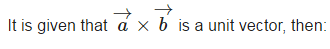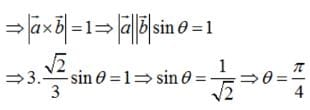Test: Vector Algebra- 2 - Question 11

The unit vector in the direction of a given vector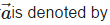Detailed Solution for Test: Vector Algebra- 2 - Question 11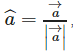is called the unit vector of a given vector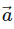Test: Vector Algebra- 2 - Question 12

Which of the following is a vector?

Detailed Solution for Test: Vector Algebra- 2 - Question 12

Acceleration is a vector quantity , because acceleration is defined as the rate of change of velocity w.r.t. time.

Test: Vector Algebra- 2 - Question 13

Find the position vector of a point R which divides the line joining two points P and Q whose position vectors are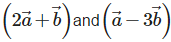externally in the ratio 1 : 2. Also, show that P is the mid point of the line segment RQ.

Detailed Solution for Test: Vector Algebra- 2 - Question 13

Let position vector of point R be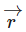. As point R divides externally the line segment PQ in the ratio 1:2 .therefore ,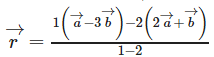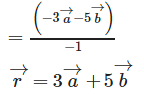Also , mid point of the line segment RQ is :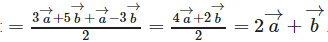Which is the position vector of point P.
Therefore , P is the mid point of line segment RQ.

Test: Vector Algebra- 2 - Question 14

Find the projection of the vector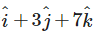on the vector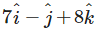Detailed Solution for Test: Vector Algebra- 2 - Question 14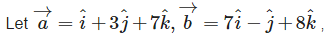then projection of vector a on vector b is given by :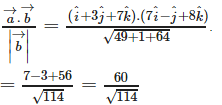Test: Vector Algebra- 2 - Question 15

If θ is the angle between two vectors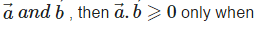Detailed Solution for Test: Vector Algebra- 2 - Question 15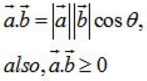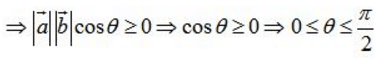Test: Vector Algebra- 2 - Question 16

Coinitial Vectors are

Detailed Solution for Test: Vector Algebra- 2 - Question 16

Two vectors whose initial point is same are called co- initial vectors

Test: Vector Algebra- 2 - Question 17

In the figure which are the Equal vectors?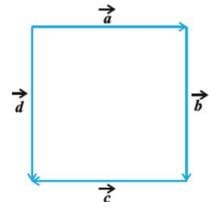Detailed Solution for Test: Vector Algebra- 2 - Question 17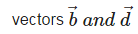are equal vectors , because they have equal magnitude and same direction.

Test: Vector Algebra- 2 - Question 18

The scalar product of two nonzero vectors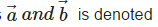Detailed Solution for Test: Vector Algebra- 2 - Question 18

The scalar product of two nonzero vectorsby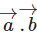Test: Vector Algebra- 2 - Question 19

Find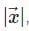if for a unit vector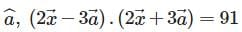Detailed Solution for Test: Vector Algebra- 2 - Question 19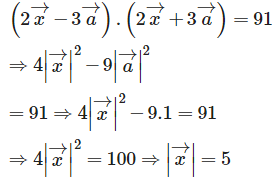Test: Vector Algebra- 2 - Question 20

If θ is the angle between any two vectors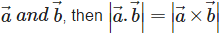when θ is equal to

Detailed Solution for Test: Vector Algebra- 2 - Question 20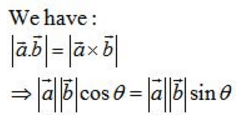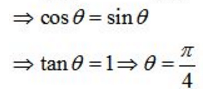Test: Vector Algebra- 2 - Question 21

Vectors A and BareCollinear

Detailed Solution for Test: Vector Algebra- 2 - Question 21

Two vectors A and B are said to be collinear , if they are parallel to the same line irrespective of their magnitudes and directions.

Test: Vector Algebra- 2 - Question 22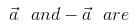Detailed Solution for Test: Vector Algebra- 2 - Question 22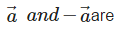collinear vectors , because they are parallel in direction and having the same magnitude.

Test: Vector Algebra- 2 - Question 23

The scalar product of two nonzero vectors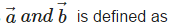Detailed Solution for Test: Vector Algebra- 2 - Question 23

The scalar product of two nonzero vectors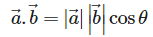Test: Vector Algebra- 2 - Question 24

If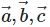are unit vectors such that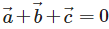then, the value of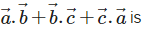Detailed Solution for Test: Vector Algebra- 2 - Question 24

It is given that :are unit vectors such thatthen :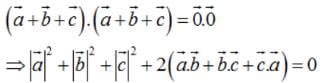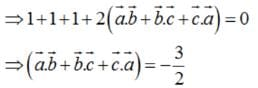Test: Vector Algebra- 2 - Question 25

Find the area of the triangle with vertices A(1, 1, 2), B(2, 3, 5) and C(1, 5, 5).

Detailed Solution for Test: Vector Algebra- 2 - Question 25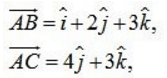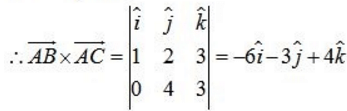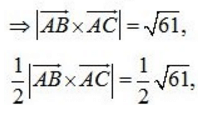Therefore , the area of triangle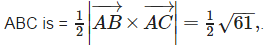## Mathematics (Maths) Class 12

206 videos|263 docs|139 tests
Information about Test: Vector Algebra- 2 Page
In this test you can find the Exam questions for Test: Vector Algebra- 2 solved & explained in the simplest way possible. Besides giving Questions and answers for Test: Vector Algebra- 2, EduRev gives you an ample number of Online tests for practice

## Mathematics (Maths) Class 12

206 videos|263 docs|139 tests